Chapter 4, Problem 4.114EP### Organic And Biological Chemistry

7th Edition
STOKER + 1 other
ISBN: 9781305081079

#### Solutions

Chapter
Section### Organic And Biological Chemistry

7th Edition
STOKER + 1 other
ISBN: 9781305081079
Textbook Problem

# Draw the condensed structural formula of a molecule with the formula C6H14O2 that fits each of the following descriptions. a. Hemiacetal of butanol b. Hemiacetal of butanone c. Acetal of butanal d. Acetal of butanone

(a)

Interpretation Introduction

Interpretation:

Condensed structural formula for the given name that has molecular formula of C6H14O2 should be drawn.

Concept Introduction:

A common nomenclature of naming acetal and hemiacetal is done by finding the product of hydrolysis of the hemiacetal or acetal.  The name of the carbonyl compound is added at the end to describe the name of such compound.

The general structure of hemiacetal is,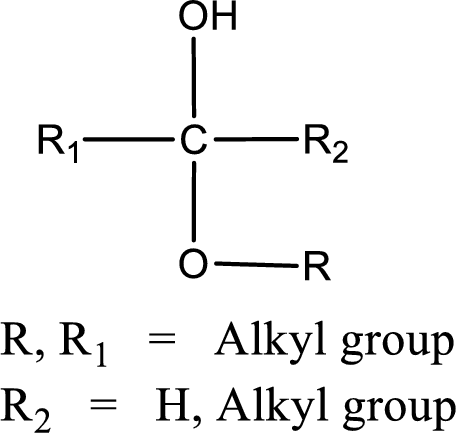The general structure of acetal is,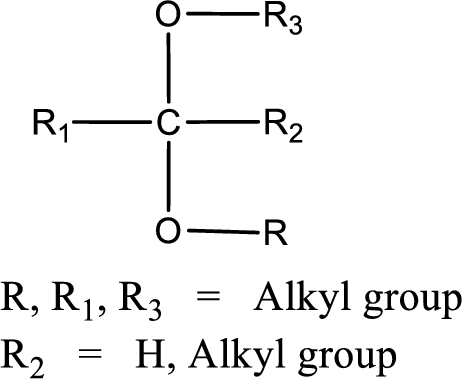Explanation

Given molecular formula of the compound is C6H14O2.  It is said that the compound is a hemiacetal of butanal

(b)

Interpretation Introduction

Interpretation:

Condensed structural formula for the given name that has molecular formula of C6H14O2 should be drawn.

Concept Introduction:

A common nomenclature of naming acetal and hemiacetal is done by finding the product of hydrolysis of the hemiacetal or acetal.  The name of the carbonyl compound is added at the end to describe the name of such compound.

The general structure of hemiacetal is,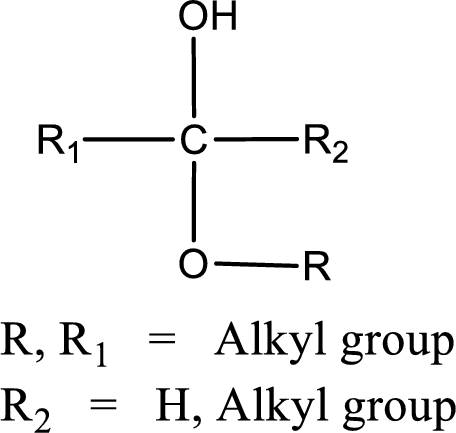The general structure of acetal is,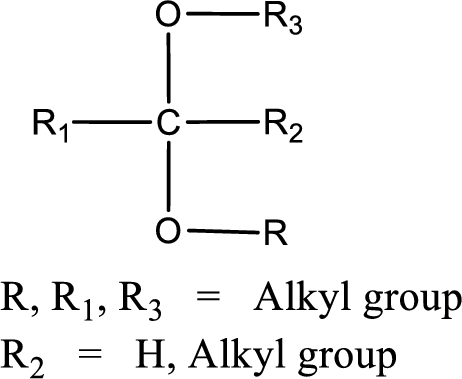(c)

Interpretation Introduction

Interpretation:

Condensed structural formula for the given name that has molecular formula of C6H14O2 should be drawn.

Concept Introduction:

A common nomenclature of naming acetal and hemiacetal is done by finding the product of hydrolysis of the hemiacetal or acetal.  The name of the carbonyl compound is added at the end to describe the name of such compound.

The general structure of hemiacetal is,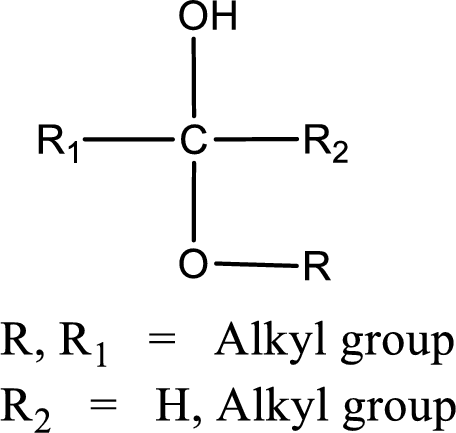The general structure of acetal is,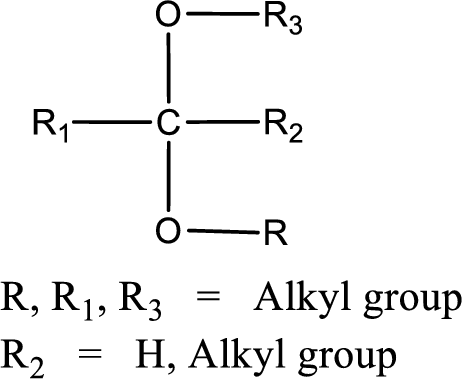(d)

Interpretation Introduction

Interpretation:

Condensed structural formula for the given name that has molecular formula of C6H14O2 should be drawn.

Concept Introduction:

A common nomenclature of naming acetal and hemiacetal is done by finding the product of hydrolysis of the hemiacetal or acetal.  The name of the carbonyl compound is added at the end to describe the name of such compound.

The general structure of hemiacetal is,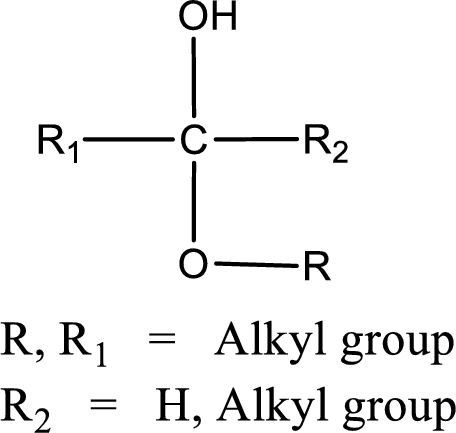The general structure of acetal is,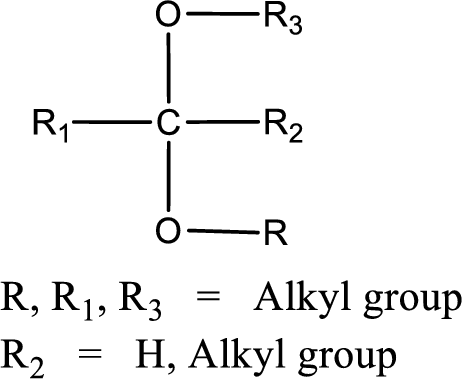### Still sussing out bartleby?

Check out a sample textbook solution.

See a sample solution

#### The Solution to Your Study Problems

Bartleby provides explanations to thousands of textbook problems written by our experts, many with advanced degrees!

Get Started